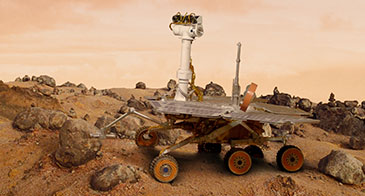Maple: A Natural Fit for Natural Convection - User Case Studies - Maplesoft﻿

# User Case Study: Maple: A Natural Fit for Natural ConvectionAt Gonzaga University, Professor Abdul Aziz teaches undergraduate mechanical engineering students about heat transfer, the study of how systems behave under the influence of heating or cooling. His students use the extensive symbolic capabilities in Maple™ to explore the principles and equations that govern natural convection without getting bogged down in complicated numerical methods.

Natural convection happens when a temperature difference causes a fluid, such as air or water, to circulate. Professor Aziz’s students learn about natural convection caused by a vertically aligned hot plate. The heat from the plate causes the surrounding air to circulate, as illustrated in Figure 1, and the students learn that the fluid motion of air, as a result of the temperature difference, can be described with two nonlinear partial differential equations. To simplify these equations into nonlinear ordinary differential equations, they use a method called “similarity solutions.” Traditionally, the results of the equations must be computed numerically since the underlying equations are nonlinear, which means that the students will know what happens, but miss out on understanding why it happens. The students get mired in generating numerical results that have little connection in their minds to the governing equations they learn in class.Figure 1. A vertical hot plate causes the air on the right to circulate, called natural convection

To address this problem, Professor Aziz turned to Maple. The powerful symbolic computational capabilities of Maple allow his students to directly manipulate the governing ordinary differential equations of natural convection. Instead of focusing on numerical calculations, they have a chance to understand all the steps involved in solving the natural convection equations. According to Professor Aziz, by using Maple, “...it is now possible to teach the students the skills necessary to solve not only the boundary layer flows but also new and challenging problems in other areas of heat transfer.” The students are also required to do their homework assignments in Maple, which has the double benefit of raising the bar on the types of problems that can be assigned and making the job of marking faster and easier.Figure 2. Dimensionless velocity profiles in natural convection boundary layers over an isothermal vertical plate at different Prandtl numbers

Maple’s ODE solvers allow the students to manipulate and simplify the governing equations, and then produce numerical results only as the final step. The built-in numerical solvers use the popular RKF45 method to produce numerical solutions to the system of equations. Since these details are mostly hidden from the user, there is no need to waste time worrying about numerical issues such as convergence and accuracy since Maple takes care of the particulars. The students can generate results for varying input parameters all using the same set of underlying equations, without the need to reconfigure the solver. Some of these results are shown in Figure 2.

Thanks to the knowledge and understanding that Maple provides in his introductory course, many students choose to continue on and take Professor Aziz’s advanced heat transfer course where they solve problems in conduction, convection, and radiation. They are able to use Maple to explore cutting-edge topics in heat transfer research. They each take on a project in such modern and diverse research areas as extended surface heat transfer, entropy generation in convection processes, convective transport in microelectromechanical systems (MEMS), similarity solutions of laminar boundary layer flows, insulation profiles for optimum heat transfer, nonlinear heat conduction, and the design and analysis of finite size radiation heat shields. Without the symbolic capabilities of Maple, it is unlikely that these advanced topics would be within the reach of undergraduate students. Now, however, Maple has taken their learning from the textbook into the real world.

Next Steps